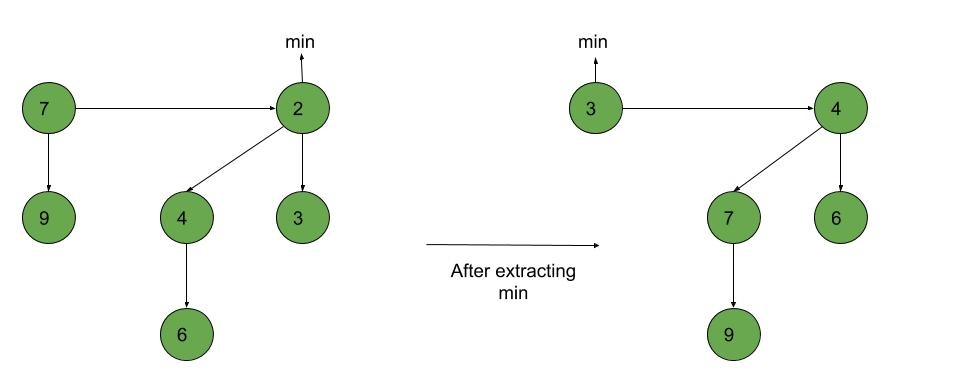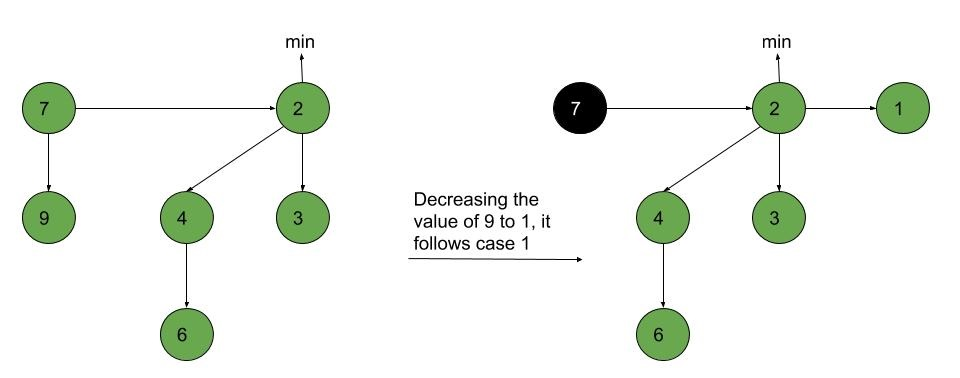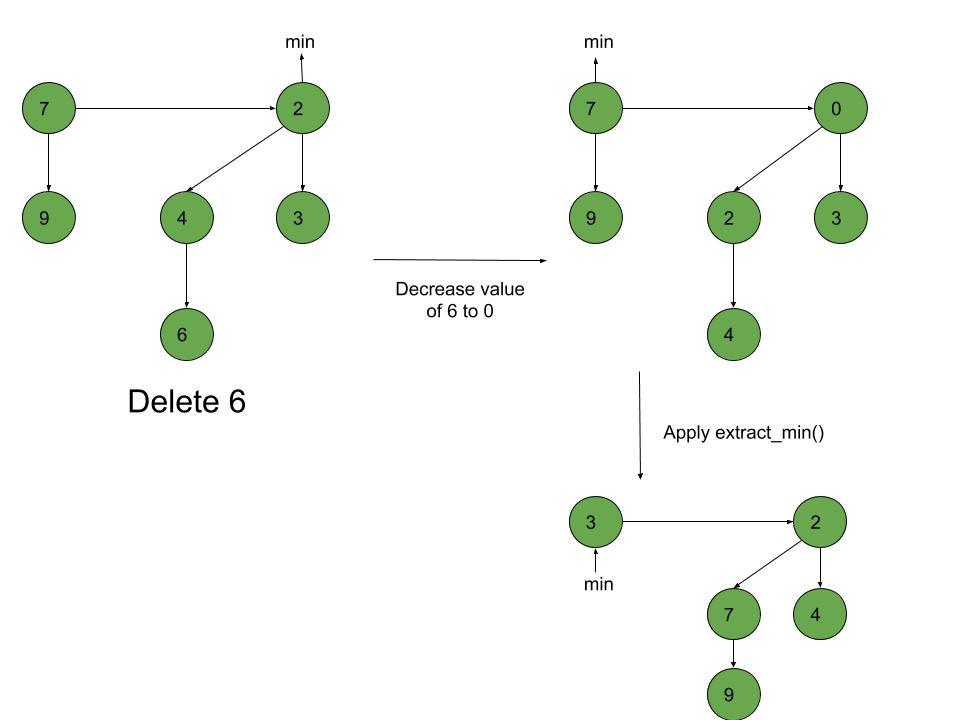# Fibonacci Heap – Deletion, Extract min and Decrease key

• Difficulty Level : Hard
• Last Updated : 06 Sep, 2022

In the last post, we discussed the Insertion and Union of Fibonacci Heaps. In this post, we will discuss Extract_min(), Decrease_key() and Deletion() operations on Fibonacci heap.

Extract_min():

We create a function for deleting the minimum node and setting the min pointer to the minimum value in the remaining heap. The following algorithm is followed:

1. Delete the min node.
2. Set head to the next min node and add all the trees of the deleted node in the root list.
3. Create an array of degree pointers of the size of the deleted node.
4. Set degree pointer to the current node.
5. Move to the next node.
• If degrees are different then set degree pointer to next node.
• If degrees are the same then join the Fibonacci trees by union operation.
6. Repeat steps 4 and 5 until the heap is completed.

Example:Decrease_key():

To decrease the value of any element in the heap, we follow the following algorithm:

• Decrease the value of the node ‘x’ to the new chosen value.
• CASE 1) If min-heap property is not violated,
• Update min pointer if necessary.
• CASE 2) If min-heap property is violated and parent of ‘x’ is unmarked,
• Cut off the link between ‘x’ and its parent.
• Mark the parent of ‘x’.
• Add tree rooted at ‘x’ to the root list and update min pointer if necessary.
• CASE 3)If min-heap property is violated and parent of ‘x’ is marked,
• Cut off the link between ‘x’ and its parent p[x].
• Add ‘x’ to the root list, updating min pointer if necessary.
• Cut off link between p[x] and p[p[x]].
• Add p[x] to the root list, updating min pointer if necessary.
• If p[p[x]] is unmarked, mark it.
• Else, cut off p[p[x]] and repeat steps 4.2 to 4.5, taking p[p[x]] as ‘x’.

Example:Deletion():

To delete any element in a Fibonacci heap, the following algorithm is followed:

1. Decrease the value of the node to be deleted ‘x’ to a minimum by Decrease_key() function.
2. By using min-heap property, heapify the heap containing ‘x’, bringing ‘x’ to the root list.
3. Apply Extract_min() algorithm to the Fibonacci heap.

Example:Following is a program to demonstrate Extract min(), Deletion() and Decrease key() operations on a Fibonacci Heap:

## C++

 `// C++ program to demonstrate Extract min, Deletion()``// and Decrease key() operations in a fibonacci heap``#include ``#include ``#include ``#include ``using` `namespace` `std;` `// Creating a structure to represent a node in the heap``struct` `node {``    ``node* parent; ``// Parent pointer``    ``node* child; ``// Child pointer``    ``node* left; ``// Pointer to the node on the left``    ``node* right; ``// Pointer to the node on the right``    ``int` `key; ``// Value of the node``    ``int` `degree; ``// Degree of the node``    ``char` `mark; ``// Black or white mark of the node``    ``char` `c; ``// Flag for assisting in the Find node function``};` `// Creating min pointer as "mini"``struct` `node* mini = NULL;` `// Declare an integer for number of nodes in the heap``int` `no_of_nodes = 0;` `// Function to insert a node in heap``void` `insertion(``int` `val)``{``    ``struct` `node* new_node = ``new` `node();``    ``new_node->key = val;``    ``new_node->degree = 0;``    ``new_node->mark = ``'W'``;``    ``new_node->c = ``'N'``;``    ``new_node->parent = NULL;``    ``new_node->child = NULL;``    ``new_node->left = new_node;``    ``new_node->right = new_node;``    ``if` `(mini != NULL) {``        ``(mini->left)->right = new_node;``        ``new_node->right = mini;``        ``new_node->left = mini->left;``        ``mini->left = new_node;``        ``if` `(new_node->key < mini->key)``            ``mini = new_node;``    ``}``    ``else` `{``        ``mini = new_node;``    ``}``    ``no_of_nodes++;``}``// Linking the heap nodes in parent child relationship``void` `Fibonnaci_link(``struct` `node* ptr2, ``struct` `node* ptr1)``{``    ``(ptr2->left)->right = ptr2->right;``    ``(ptr2->right)->left = ptr2->left;``    ``if` `(ptr1->right == ptr1)``        ``mini = ptr1;``    ``ptr2->left = ptr2;``    ``ptr2->right = ptr2;``    ``ptr2->parent = ptr1;``    ``if` `(ptr1->child == NULL)``        ``ptr1->child = ptr2;``    ``ptr2->right = ptr1->child;``    ``ptr2->left = (ptr1->child)->left;``    ``((ptr1->child)->left)->right = ptr2;``    ``(ptr1->child)->left = ptr2;``    ``if` `(ptr2->key < (ptr1->child)->key)``        ``ptr1->child = ptr2;``    ``ptr1->degree++;``}``// Consolidating the heap``void` `Consolidate()``{``    ``int` `temp1;``    ``float` `temp2 = (``log``(no_of_nodes)) / (``log``(2));``    ``int` `temp3 = temp2;``    ``struct` `node* arr[temp3+1];``    ``for` `(``int` `i = 0; i <= temp3; i++)``        ``arr[i] = NULL;``    ``node* ptr1 = mini;``    ``node* ptr2;``    ``node* ptr3;``    ``node* ptr4 = ptr1;``    ``do` `{``        ``ptr4 = ptr4->right;``        ``temp1 = ptr1->degree;``        ``while` `(arr[temp1] != NULL) {``            ``ptr2 = arr[temp1];``            ``if` `(ptr1->key > ptr2->key) {``                ``ptr3 = ptr1;``                ``ptr1 = ptr2;``                ``ptr2 = ptr3;``            ``}``            ``if` `(ptr2 == mini)``                ``mini = ptr1;``            ``Fibonnaci_link(ptr2, ptr1);``            ``if` `(ptr1->right == ptr1)``                ``mini = ptr1;``            ``arr[temp1] = NULL;``            ``temp1++;``        ``}``        ``arr[temp1] = ptr1;``        ``ptr1 = ptr1->right;``    ``} ``while` `(ptr1 != mini);``    ``mini = NULL;``    ``for` `(``int` `j = 0; j <= temp3; j++) {``        ``if` `(arr[j] != NULL) {``            ``arr[j]->left = arr[j];``            ``arr[j]->right = arr[j];``            ``if` `(mini != NULL) {``                ``(mini->left)->right = arr[j];``                ``arr[j]->right = mini;``                ``arr[j]->left = mini->left;``                ``mini->left = arr[j];``                ``if` `(arr[j]->key < mini->key)``                    ``mini = arr[j];``            ``}``            ``else` `{``                ``mini = arr[j];``            ``}``            ``if` `(mini == NULL)``                ``mini = arr[j];``            ``else` `if` `(arr[j]->key < mini->key)``                ``mini = arr[j];``        ``}``    ``}``}` `// Function to extract minimum node in the heap``void` `Extract_min()``{``    ``if` `(mini == NULL)``        ``cout << ``"The heap is empty"` `<< endl;``    ``else` `{``        ``node* temp = mini;``        ``node* pntr;``        ``pntr = temp;``        ``node* x = NULL;``        ``if` `(temp->child != NULL) {` `            ``x = temp->child;``            ``do` `{``                ``pntr = x->right;``                ``(mini->left)->right = x;``                ``x->right = mini;``                ``x->left = mini->left;``                ``mini->left = x;``                ``if` `(x->key < mini->key)``                    ``mini = x;``                ``x->parent = NULL;``                ``x = pntr;``            ``} ``while` `(pntr != temp->child);``        ``}``        ``(temp->left)->right = temp->right;``        ``(temp->right)->left = temp->left;``        ``mini = temp->right;``        ``if` `(temp == temp->right && temp->child == NULL)``            ``mini = NULL;``        ``else` `{``            ``mini = temp->right;``            ``Consolidate();``        ``}``        ``no_of_nodes--;``    ``}``}` `// Cutting a node in the heap to be placed in the root list``void` `Cut(``struct` `node* found, ``struct` `node* temp)``{``    ``if` `(found == found->right)``        ``temp->child = NULL;` `    ``(found->left)->right = found->right;``    ``(found->right)->left = found->left;``    ``if` `(found == temp->child)``        ``temp->child = found->right;` `    ``temp->degree = temp->degree - 1;``    ``found->right = found;``    ``found->left = found;``    ``(mini->left)->right = found;``    ``found->right = mini;``    ``found->left = mini->left;``    ``mini->left = found;``    ``found->parent = NULL;``    ``found->mark = ``'B'``;``}` `// Recursive cascade cutting function``void` `Cascase_cut(``struct` `node* temp)``{``    ``node* ptr5 = temp->parent;``    ``if` `(ptr5 != NULL) {``        ``if` `(temp->mark == ``'W'``) {``            ``temp->mark = ``'B'``;``        ``}``        ``else` `{``            ``Cut(temp, ptr5);``            ``Cascase_cut(ptr5);``        ``}``    ``}``}` `// Function to decrease the value of a node in the heap``void` `Decrease_key(``struct` `node* found, ``int` `val)``{``    ``if` `(mini == NULL)``        ``cout << ``"The Heap is Empty"` `<< endl;` `    ``if` `(found == NULL)``        ``cout << ``"Node not found in the Heap"` `<< endl;` `    ``found->key = val;` `    ``struct` `node* temp = found->parent;``    ``if` `(temp != NULL && found->key < temp->key) {``        ``Cut(found, temp);``        ``Cascase_cut(temp);``    ``}``    ``if` `(found->key < mini->key)``        ``mini = found;``}` `// Function to find the given node``void` `Find(``struct` `node* mini, ``int` `old_val, ``int` `val)``{``    ``struct` `node* found = NULL;``    ``node* temp5 = mini;``    ``temp5->c = ``'Y'``;``    ``node* found_ptr = NULL;``    ``if` `(temp5->key == old_val) {``        ``found_ptr = temp5;``        ``temp5->c = ``'N'``;``        ``found = found_ptr;``        ``Decrease_key(found, val);``    ``}``    ``if` `(found_ptr == NULL) {``        ``if` `(temp5->child != NULL)``            ``Find(temp5->child, old_val, val);``        ``if` `((temp5->right)->c != ``'Y'``)``            ``Find(temp5->right, old_val, val);``    ``}``    ``temp5->c = ``'N'``;``    ``found = found_ptr;``}` `// Deleting a node from the heap``void` `Deletion(``int` `val)``{``    ``if` `(mini == NULL)``        ``cout << ``"The heap is empty"` `<< endl;``    ``else` `{` `        ``// Decreasing the value of the node to 0``        ``Find(mini, val, 0);` `        ``// Calling Extract_min function to``        ``// delete minimum value node, which is 0``        ``Extract_min();``        ``cout << ``"Key Deleted"` `<< endl;``    ``}``}` `// Function to display the heap``void` `display()``{``    ``node* ptr = mini;``    ``if` `(ptr == NULL)``        ``cout << ``"The Heap is Empty"` `<< endl;` `    ``else` `{``        ``cout << ``"The root nodes of Heap are: "` `<< endl;``        ``do` `{``            ``cout << ptr->key;``            ``ptr = ptr->right;``            ``if` `(ptr != mini) {``                ``cout << ``"-->"``;``            ``}``        ``} ``while` `(ptr != mini && ptr->right != NULL);``        ``cout << endl``             ``<< ``"The heap has "` `<< no_of_nodes << ``" nodes"` `<< endl``             ``<< endl;``    ``}``}` `// Driver code``int` `main()``{``    ``// We will create a heap and insert 3 nodes into it``    ``cout << ``"Creating an initial heap"` `<< endl;``    ``insertion(5);``    ``insertion(2);``    ``insertion(8);` `    ``// Now we will display the root list of the heap``    ``display();` `    ``// Now we will extract the minimum value node from the heap``    ``cout << ``"Extracting min"` `<< endl;``    ``Extract_min();``    ``display();` `    ``// Now we will decrease the value of node '8' to '7'``    ``cout << ``"Decrease value of 8 to 7"` `<< endl;``    ``Find(mini, 8, 7);``    ``display();` `    ``// Now we will delete the node '7'``    ``cout << ``"Delete the node 7"` `<< endl;``    ``Deletion(7);``    ``display();` `    ``return` `0;``}`

## Python3

 `# Python3 program to demonstrate Extract min, Deletion()``# and Decrease key() operations in a fibonacci heap``import` `math` `# Creating a class to represent a node in the heap``class` `node:``    ``def` `__init__(``self``):``        ``parent``=``None` `# Parent pointer``        ``child``=``None` `# Child pointer``        ``left``=``None` `# Pointer to the node on the left``        ``right``=``None` `# Pointer to the node on the right``        ``key``=``-``1` `# Value of the node``        ``degree``=``-``1` `# Degree of the node``        ``mark``=``'' ``# Black or white mark of the node``        ``c``=``'' ``# Flag for assisting in the Find node function` `# Creating min pointer as "mini"``mini ``=` `None` `# Declare an integer for number of nodes in the heap``no_of_nodes ``=` `0` `# Function to insert a node in heap``def` `insertion(val):``    ``global` `mini,no_of_nodes` `    ``new_node ``=` `node()``    ``new_node.key ``=` `val``    ``new_node.degree ``=` `0``    ``new_node.mark ``=` `'W'``    ``new_node.c ``=` `'N'``    ``new_node.parent ``=` `None``    ``new_node.child ``=` `None``    ``new_node.left ``=` `new_node``    ``new_node.right ``=` `new_node``    ``if` `(mini !``=` `None``):``        ``mini.left.right ``=` `new_node``        ``new_node.right ``=` `mini``        ``new_node.left ``=` `mini.left``        ``mini.left ``=` `new_node``        ``if` `(new_node.key < mini.key):``            ``mini ``=` `new_node``    ``else``:``        ``mini ``=` `new_node``    ``no_of_nodes``+``=``1` `#  Linking the heap nodes in parent child relationship``def` `Fibonnaci_link(ptr2, ptr1):``    ``ptr2.left.right ``=` `ptr2.right``    ``ptr2.right.left ``=` `ptr2.left``    ``if` `(ptr1.right ``=``=` `ptr1):``        ``mini ``=` `ptr1``    ``ptr2.left ``=` `ptr2``    ``ptr2.right ``=` `ptr2``    ``ptr2.parent ``=` `ptr1``    ``if` `(ptr1.child ``=``=` `None``):``        ``ptr1.child ``=` `ptr2``    ``ptr2.right ``=` `ptr1.child``    ``ptr2.left ``=` `ptr1.child.left``    ``ptr1.child.left.right ``=` `ptr2``    ``ptr1.child.left ``=` `ptr2``    ``if` `ptr2.key < ptr1.child.key:``        ``ptr1.child ``=` `ptr2``    ``ptr1.degree``+``=``1` `# Consolidating the heap``def` `Consolidate():``    ` `    ``global` `mini``    ``temp2 ``=` `math.log2(no_of_nodes)``    ``temp3 ``=` `int``(temp2)``    ``arr``=``[``None``]``*``(temp3``+``1``)``    ``for` `i ``in` `range``(temp3``+``1``):``        ``arr[i] ``=` `None``    ``ptr1 ``=` `mini``    ``ptr4 ``=` `ptr1``    ``while` `True``:``        ``ptr4 ``=` `ptr4.right``        ``temp1 ``=` `ptr1.degree``        ``while` `(arr[temp1] !``=` `None``):``            ``ptr2 ``=` `arr[temp1]``            ``if` `(ptr1.key > ptr2.key):``                ``ptr3 ``=` `ptr1``                ``ptr1 ``=` `ptr2``                ``ptr2 ``=` `ptr3``            ``if` `(ptr2 ``=``=` `mini):``                ``mini ``=` `ptr1``            ``Fibonnaci_link(ptr2, ptr1)``            ``if` `(ptr1.right ``=``=` `ptr1):``                ``mini ``=` `ptr1``            ``arr[temp1] ``=` `None``            ``temp1``+``=``1``        ``arr[temp1] ``=` `ptr1``        ``ptr1 ``=` `ptr1.right``        ``if` `(ptr1 ``=``=` `mini):``            ``break``    ``mini ``=` `None``    ``for` `j ``in` `range``(temp3``+``1``):``        ``if` `(arr[j] !``=` `None``):``            ``arr[j].left ``=` `arr[j]``            ``arr[j].right ``=` `arr[j]``            ``if` `(mini !``=` `None``) :``                ``mini.left.right ``=` `arr[j]``                ``arr[j].right ``=` `mini``                ``arr[j].left ``=` `mini.left``                ``mini.left ``=` `arr[j]``                ``if` `(arr[j].key < mini.key):``                    ``mini ``=` `arr[j]``            ``else``:``                ``mini ``=` `arr[j]``            ``if` `mini ``=``=` `None``:``                ``mini ``=` `arr[j]``            ``elif` `arr[j].key < mini.key:``                ``mini ``=` `arr[j]``    `  `# Function to extract minimum node in the heap``def` `Extract_min():` `    ``global` `mini,no_of_nodes``    ``if` `mini ``=``=` `None``:``        ``print``(``"The heap is empty"``)``    ``else``:``        ``temp ``=` `mini``        ``pntr ``=` `temp``        ``x ``=` `None``        ``if` `(temp.child !``=` `None``):` `            ``x ``=` `temp.child``            ``while``(``True``):``                ``pntr ``=` `x.right``                ``mini.left.right ``=` `x``                ``x.right ``=` `mini``                ``x.left ``=` `mini.left``                ``mini.left ``=` `x``                ``if` `x.key < mini.key:``                    ``mini ``=` `x``                ``x.parent ``=` `None``                ``x ``=` `pntr``                ``if` `(pntr ``=``=` `temp.child):``                    ``break` `        ``temp.left.right ``=` `temp.right``        ``temp.right.left ``=` `temp.left``        ``mini ``=` `temp.right``        ``if` `temp ``=``=` `temp.right ``and` `temp.child ``=``=` `None``:``            ``mini ``=` `None``        ``else``:``            ``mini ``=` `temp.right``            ``Consolidate()``        ``no_of_nodes``-``=``1`   `# Cutting a node in the heap to be placed in the root list``def` `Cut(found, temp):` `    ``if` `(found ``=``=` `found.right):``        ``temp.child ``=` `None` `    ``found.left.right ``=` `found.right``    ``found.right.left ``=` `found.left``    ``if` `(found ``=``=` `temp.child):``        ``temp.child ``=` `found.right` `    ``temp.degree ``=` `temp.degree ``-` `1``    ``found.right ``=` `found``    ``found.left ``=` `found``    ``mini.left.right ``=` `found``    ``found.right ``=` `mini``    ``found.left ``=` `mini.left``    ``mini.left ``=` `found``    ``found.parent ``=` `None``    ``found.mark ``=` `'B'` `# Recursive cascade cutting function``def` `Cascase_cut(temp):` `    ``ptr5 ``=` `temp.parent``    ``if` `(ptr5 !``=` `None``):``        ``if` `(temp.mark ``=``=` `'W'``):``            ``temp.mark ``=` `'B'``        ``else``:``            ``Cut(temp, ptr5)``            ``Cascase_cut(ptr5)` `# Function to decrease the value of a node in the heap``def` `Decrease_key(found, val):` `    ``global` `mini``    ``if` `(mini ``=``=` `None``):``        ``print``(``"The Heap is Empty"``)` `    ``if` `found ``=``=` `None``:``        ``print``(``"Node not found in the Heap"``)` `    ``found.key ``=` `val` `    ``temp ``=` `found.parent``    ``if` `(temp !``=` `None` `and` `found.key < temp.key):``        ``Cut(found, temp)``        ``Cascase_cut(temp)` `    ``if` `(found.key < mini.key):``        ``mini ``=` `found`  `# Function to find the given node``def` `Find(mini, old_val, val):` `    ``found ``=` `None``    ``temp5 ``=` `mini``    ``temp5.c ``=` `'Y'``    ``found_ptr ``=` `None``    ``if` `(temp5.key ``=``=` `old_val):``        ``found_ptr ``=` `temp5``        ``temp5.c ``=` `'N'``        ``found ``=` `found_ptr``        ``Decrease_key(found, val)` `    ``if` `(found_ptr ``=``=` `None``):``        ``if` `(temp5.child !``=` `None``):``            ``Find(temp5.child, old_val, val)``        ``if` `temp5.right.c !``=` `'Y'``:``            ``Find(temp5.right, old_val, val)``    ``temp5.c ``=` `'N'``    ``found ``=` `found_ptr`  `# Deleting a node from the heap``def` `Deletion(val):` `    ``if` `(mini ``=``=` `None``):``        ``print``(``"The heap is empty"``)``    ``else``:``        ``# Decreasing the value of the node to 0``        ``Find(mini, val, ``0``)` `        ``# Calling Extract_min function to``        ``# delete minimum value node, which is 0``        ``Extract_min()``        ``print``(``"Key Deleted"``)`  `# Function to display the heap``def` `display():``    ``ptr ``=` `mini``    ``if` `(ptr ``=``=` `None``):``        ``print``(``"The Heap is Empty"``)` `    ``else``:``        ``print``(``"The root nodes of Heap are: "``)``        ``while``(``True``):``            ``print``(ptr.key,end``=``'')``            ``ptr ``=` `ptr.right``            ``if` `(ptr !``=` `mini):``                ``print``(``"-->"``,end``=``'')``            ``if` `not``(ptr !``=` `mini ``and` `ptr.right !``=` `None``):``                ``break``        ``print``()``        ``print``(``"The heap has {} nodes"``.``format``(no_of_nodes))` `# Driver code``if` `__name__ ``=``=` `'__main__'``:` `    ``# We will create a heap and insert 3 nodes into it``    ``print``(``"Creating an initial heap"``)``    ``insertion(``5``)``    ``insertion(``2``)``    ``insertion(``8``)` `    ``# Now we will display the root list of the heap``    ``display()` `    ``# Now we will extract the minimum value node from the heap``    ``print``(``"Extracting min"``)``    ``Extract_min()``    ``display()` `    ``# Now we will decrease the value of node '8' to '7'``    ``print``(``"Decrease value of 8 to 7"``)``    ``Find(mini, ``8``, ``7``)``    ``display()` `    ``print``(``"Now we will delete the node '7'"``)``    ``print``(``"Delete the node 7"``)``    ``Deletion(``7``)``    ``display()` `# This code is contributed by Amartya Ghosh`

Output:

```Creating an initial heap
The root nodes of Heap are:
2-->5-->8
The heap has 3 nodes

Extracting min
The root nodes of Heap are:
5
The heap has 2 nodes

Decrease value of 8 to 7
The root nodes of Heap are:
5
The heap has 2 nodes

Delete the node 7
Key Deleted
The root nodes of Heap are:
5
The heap has 1 nodes```

My Personal Notes arrow_drop_up